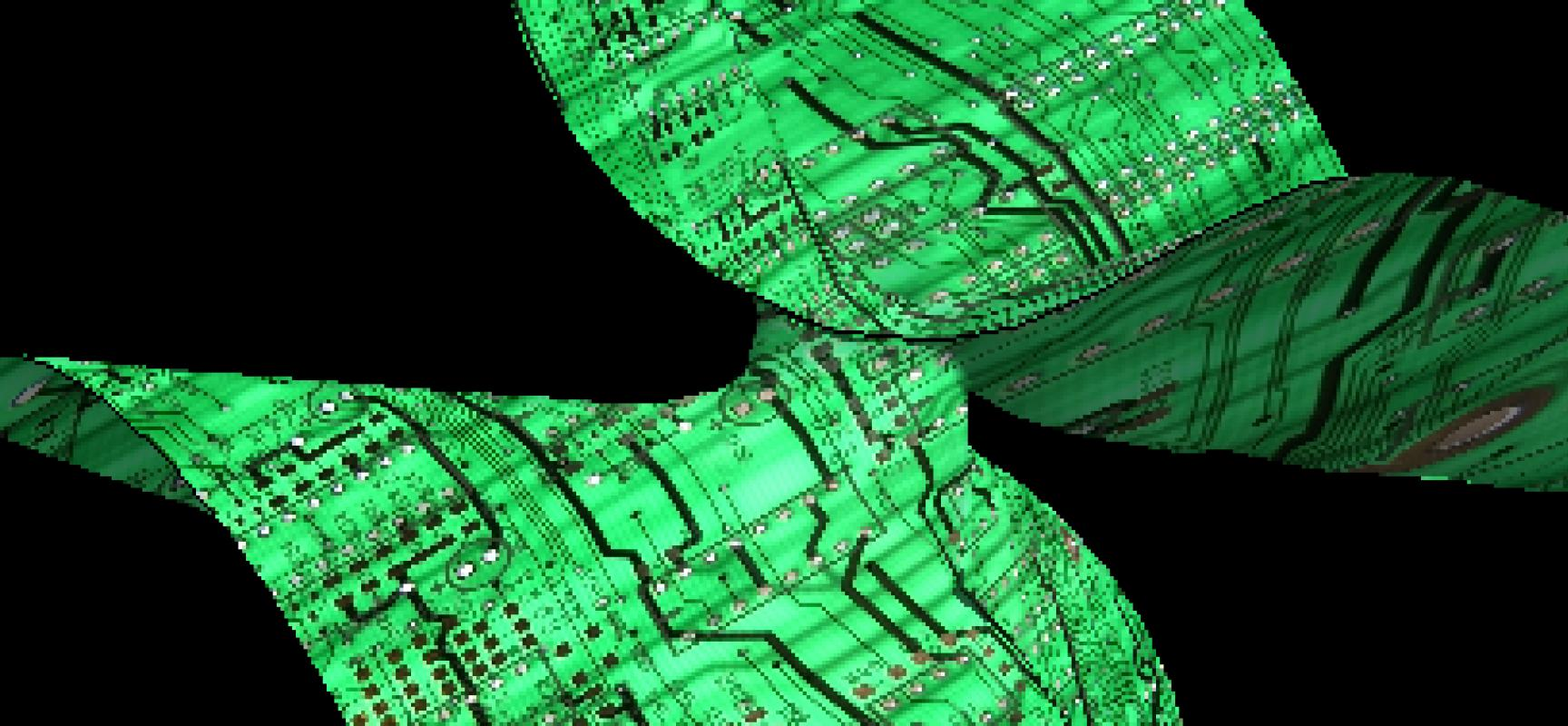# From the Inside: Algorithms and Complexity in Algebraic GeometryBy JM Landsberg

Nearly all scientists use linear algebra and recognize its importance. The utility of algebraic computation beyond linear algebra is just beginning to be recognized and exploited. Algebraic geometry studies the zero sets of collections of polynomials, both quantitatively and qualitatively. It is one of the oldest areas of mathematics. In the second half of the last century, algebraic geometers developed abstract language that enabled them to solve questions that had been open for hundreds of years.

An unfortunate byproduct of this language was that, despite its utility, the subject became inaccessible to researchers outside the field. Recently, however, there has been an explosion of activity, as physicists, applied mathematicians, computer scientists, engineers, and other researchers have realized its utility, and at the same time geometers have reached out to understand their questions. The central goal of the semester is to develop deep and long-lasting exchanges and collaborations between algebraic geometers and computer scientists.

Two broad themes of the semester are non-linear questions in computational geometry and proving upper and lower bounds in complexity theory.

Regarding computational geometry, basic questions in diverse areas such as physics, signal processing, statistics, and biology can naturally be phrased within the framework of algebraic geometry. Advances in computer science have spawned the field of computational algebraic geometry, which has led to the development and implementation of new, efficient algorithms. Several old and new methods from algebraic geometry have led to significant and unexpected advances in the above-mentioned areas of applications. These advances (e.g., the effective Nullstellensatz and its consequences) are intertwined with the study of the complexity of solving basic computational problems in algebraic geometry.

Returning to linear algebra, the basic operation of linear algebra is matrix multiplication. The standard algorithm to multiply n × n matrices uses on the order of n3 arithmetic operations. In 1969, V. Strassen realized that matrices could be multiplied using on the order of n2.81 arithmetic operations, which inspired substantial research that led computer scientists to make the astounding conjecture that asymptotically it is not much harder to multiply matrices than it is to add them! Leaders in this area, including V. Williams, will work with algebraic geometers to attempt to resolve this conjecture.

In contrast to the progress on upper bounds, lower bounds have been difficult for complexity theorists. For example, chapter 14 of Computational complexity: a modern approach (Arora and Barak, Cambridge Univ. Press) is "Circuit lower bounds: Complexity theory's Waterloo". Another goal of the semester is to change this situation. An early example of the use of algebraic geometry for lower bounds is Strassen's discovery of algebraic invariants that proved super-linear complexity lower bounds for arithmetic circuits. The recent best known lower bound for the border rank of matrix multiplication builds on Strassen's work, while using more advanced techniques from geometry and representation theory.

The geometric complexity theory program concerns the permanent versus determinant question. Among the fundamental problems inspired by Gödel's attempt to quantify the notion of intuition and the question of whether problems that admit a fast verification also necessarily admit fast solutions, it is perhaps the only program with an (admittedly difficult) road map towards a resolution. The permanent versus determinant problem (essentially VP vs VNP) as well as the complexity of matrix multiplication, can be naturally restated in the framework of algebraic geometry as explicit orbit closure problems.

The program has a diverse collection of participants, including algebraic geometers, complexity theorists, and physicists. It includes a boot camp and four workshops:

The boot camp (Sept. 2–5) served to introduce the themes of the semester to participants. The speakers were a mixture of senior scientists and young researchers.

Geometric Complexity Theory (Sept. 15–19) addressed fundamental complexity lower bound questions such as P versus NP by means of algebraic geometry and representation theory.

Solving Polynomial Equations (Oct. 13–16) which will focus on recent algorithmic advances, both numeric and symbolic, on novel domains of application, and on fundamental issues of complexity in algebraic geometry.

Tensors in Computer Science and Geometry (Nov. 10–14) will focus on the latest algorithmic, complexity, and mathematical results in multilinear algebra, with a view towards questions of relevance in computer science.

Symbolic and Numerical Methods for Tensors and Representation Theory (Nov. 17–20) will address theoretical and computational topics, with an emphasis on open problems, as well as sessions on coding and experimentation with the computer algebra system Macaulay2.

,